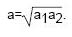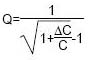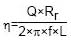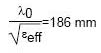?
EE Times-Asia > RF/Microwave
?
?
RF/Microwave??# Fundamentals and design principles of RF, antenna (Part 3)

Posted: 14 Mar 2008 ?? ?Print Version ?

Keywords:single loop antenna? PCB? resistor?

By Matthew Loy and Iboun Sylla
Texas Instruments Inc.

Antennas connect RF signals in an electrical circuit, such as between a PCB and an electromagnetic wave propagating in the transmission media between the transmitter and the receiver of a wireless link.

The conclusion of the three-part series focuses on small loop antennas with differential feed, single-ended loop and trapped PCB loop antennas.

The loop antenna shown in Figure 13 has a differential feed. Often a ground plane is made part of the loop, giving a single-ended feed as shown in Figure 14.

The small arrows indicate the current flow through the loop. On the ground plane, the current is mainly concentrated on the surface. The electrical behavior of the structure in Figure 14 is therefore similar to that of the loop with differential feed shown in Figure 13.

The following considerations on small loop antennas are based on and assume that the current is constant over the loop. This means that the circumference must be smaller than one tenth of a wavelength. Although this is rarely the case, the given formulas describe the principal behavior and can be used as a starting point for the loop antenna design.

If the current is constant over the loop, we can consider the loop as a radiating inductor with inductance L, where L is the inductance of the wire or PCB trace. Together with the capacitor C, this inductance L builds a resonant circuit. Often a resistor Ratt is added to reduce the quality factor of the antenna and to make it less sensitive to tolerances. Of course, this resistor dissipates energy and reduces the antenna's efficiency.

The following calculations hold for circular loops with the radius a for square loops with the side length a. A rectangular antenna with the sides a1 and a will be approximated by an equivalent square with the side length:The length (circumference) of the wire building the loop will be called U, where U = 2πa for a circular loop, or 4a for a square loop.

For the calculation of the inductance, the wire radius b, where b is 1/2 the diameter of the actual wire used to fabricate the antenna, is needed. In the frequent case where a loop antenna is realized by a trace on a PCB, b = 0.35.d + 0.24.w can be used, where d is the thickness of the copper layer and w is the trace width.

Figure 15 shows the equivalent schematic of a small loop antenna.The radiation resistance of loop antennas is small, typically smaller than 1?. The loss resistance Rloss describes the ohmic losses in the loop conductor and the losses in the capacitor (expressed by its ESR). Usually, the ESR of the capacitor cannot be neglected. Interestingly, the thickness of the copper foil is not needed for the calculation of the loss resistance because due to the skin effect, the current is confined on the conductor surface.Figure 13: Small loop antenna with differential feed

Together with the loop inductance L, which is the inductance of the wire, the capacitor C builds a series resonant circuit. In practice, the L-to-C ratio of this resonant circuit is large giving a high quality factor (Q). This would make the antenna sensitive to tolerances. That's why often an attenuating resistor Ratt is added to reduce the Q. To describe the influence of Ratt on the loop antenna, we make a parallel to series conversion and use the equivalent series resistance Ratt trans. The resistance value of Ratt trans is determined by the acceptable tolerance of the capacitor and the geometry of the loop.Figure 14: Single-ended loop antenna

The maximum usable quality factor is calculated from the capacitance tolerances ΔC/C:The series transformed attenuation resistance then will be:This gives the efficiency of the loop antenna:In most cases, the radiation resistance is much smaller than the loss resistance and the transformed attenuation resistance, giving a poor efficiency. In this case, the approximation:can be used. Rr is determined by the loop area, which is πa2 for circular loops, a2 for square loops, and a1a2 for rectangular loops. Figure 16 shows the efficiency of small circular loop antennas versus their diameter for an assumed tolerance of 5 percent. The trace width has been assumed as 1 mm, the copper thickness as 50 um; but both values have only a minor influence on the efficiency, which is mainly determined by the attenuation resistance Ratt. As expected, the efficiency increases with increasing diameter.Figure 15: Equivalent schematics of the small loop antenna in Figure 13Figure 16: Efficiency of small loop antennas for five-percent tolerance

If we feed the loop antenna as shown in Figure 14, the series equivalent circuit of Figure 15 describes the antenna impedance. Even including the effect of Ratt, the total series resistance will be small, usually below 10?. If we feed the antenna directly at the capacitor, the parallel equivalent circuit describes the antenna impedance. A small series loss resistor transforms into a large parallel resistor, usually several k?s.

In both cases, matching to 50? will be difficult. That's why the loop antenna is often tapped, giving an impedance in between the too small and the too large values. Figure 17 shows an example:Figure 17: Example of a tapped PCB loop antenna

A series feed (in the lower right corner) would give a small impedance. A parallel feed (directly at the capacitors) would give a much too large impedance. The tap provides an impedance close to 50? in this example. The loop capacitor has been split into two series capacitors C1 and C2. This makes it possible to realize non-IEC capacitance values. R1 is the damping resistor which de-Qs the circuit, thus increasing the bandwidth and subsequently reducing the tolerance requirements.

Unfortunately, there are no easy formulas that describe the tapped structure and give the right location for the tap. The line from the antenna termination to the tap is not a transmission line and will disturb the field in the antenna. Therefore, we have to find out the optimal structure by electromagnetic simulations. Often a trial and error procedure is used as an alternative. For example, using a vector network analyzer, we determine the capacitance value that gives the best return loss and the largest resistance value that gives the required bandwidth.

The loop antenna gives a linear polarization with the vector of the electric field oscillating in the plane built by the loop.

In contrast to all of the antennas discussed so far, loop antennas are magnetic antennas. This means that they are not detuned by the dielectric constant of the material in their reactive near field. That's why loop antennas are often used for body-worn or hand-held equipment.

Table 2 shows a feature comparison of the discussed antennas.Antenna design rules
We can summarize the considerations made so far in the following rules of thumb:

• If the available space is sufficient, use a half-wave dipole (for differential feed) or quarter-wave monopole (for single-ended feed) antenna for best efficiency.

• If possible, keep the space around the antenna clear from conducting or dielectric materials, such as electronic components, the casing or the user's body.

• Sometimes, dielectric materials in the reactive near field are unavoidable. In these cases, measure the antenna impedance under real application conditions and match it to the needed characteristic impedance.

• Due to space limitations, the ground plane of quarter-wave monopoles is often too small. In these cases try to create as much ground plane around the feed point as possible, measure the resulting antenna impedance and match it to the needed characteristic impedance. Good performance can be obtained from a counterpoise made from a quarter-wavelength conductor that is connected to ground in the vicinity of the antenna's feeding point. The counterpoise should run as long as possible perpendicular to the monopole radiator.

• When using premanufactured antennas, keep in mind that their performance depends on the attached ground plane. The manufacturer's specifications are only achieved if the ground plane has the same size and shape as the manufacturer's evaluation board. In all other cases, you have to measure the impedance of the premanufactured antenna under application conditions and to match it to the needed characteristic impedance.

• Small loop antennas are insensitive to varying dielectric conditions in their reactive near field. They can be a good solution for portable and hand-held devices but have a much lower efficiency than electrical antennas. Only antennas with a circumference smaller than one tenth of a wavelength can be considered as purely magnetic antennas. Larger loops have a higher gain but also a higher sensitivity to the environmental conditions.

• Size matters: Always keep in mind that Chu's and Wheeler's limit determines the product of the bandwidth and the efficiency for a given antenna dimension. An extremely small antenna cannot be efficient and tolerance-insensitive at the same time.

TRF6901 ref design
Figure 18 shows the structure of the antenna used in the TRF6901 reference design:Figure 17: PCB antenna layout on the TRF6901 reference design

Beginning from the feeding point, two legs with different lengths run in parallel to the PCB ground plane and can be terminated at their ends by SMD components to ground. Depending on the used termination elements the layout can be used as an inverted-F antenna or a tapped small loop antenna.

The short leg is usually terminated by a 0? resistor. If the long arm is left open, we get an inverted-F antenna. Terminating the long arm with a capacitor and a resistor in parallel gives a tapped loop antenna similar to the one shown in Figure 17; 1 k? and 0.5pF bring the loop into resonance at 915MHz.

When the antenna is used as a loop antenna, we have to consider the circumference of the loop in relation to the wavelength on the PCB. The trace width of the antenna in the reference design is 1.5mm and the thickness of the PCB material is also 1.5mm. As an approximation, we calculate the effective dielectric constant according to Section 2.2 and get εeff = 3.1. The wavelength of a 915MHz signal on the PCB is then:The circumference of the loop in the reference design is 91mm, which is almost half a wavelength. The antenna behavior will therefore be different from that of a pure magnetic antenna; the antenna will excite some electrical field in the reactive near field too. This gives a gain larger than predicted for the loop antenna in Figure 17.

As we can see from Figure 18, the antenna is bent around the corner of the PCB. This gives radiation in both the x and y direction and helps to make the radiation pattern more omni directional.

References
L.J.Chu, Physical limitations of Omni-Directional Antennas, J. Appl. Phys., Vol19, Dec. 1948, pp. 1163-1175
H.A.Wheeler, Fundamental Limitations of Small Antennas, Proc. IRE>, Vol. 35, Dec. 1947, pp. 1479-1484

Fundamentals and design principles of RF, antenna (Part 1)
Fundamentals and design principles of RF, antenna (Part 2)

?
?
WebinarsVisit Asia Webinars to learn about the latest in technology and get practical design tips.

?
?